Light on Math Machine Learning: Intuitive Guide to Neural Style Transfer

Introduction

Neural style transfer (NST) is a very neat idea. NST builds on the key idea that,

it is possible to separate the style representation and content representations in a CNN, learnt during a computer vision task (e.g. image recognition task).

Following this concept, NST employs a pretrained convolution neural network (CNN) to transfer styles from a given image to another. This is done by defining a loss function that tries to minimise the differences between a content image, a style image and a generated image, which will be discussed in detail later. By the end of this tutorial you will be able to create very cool artwork like below.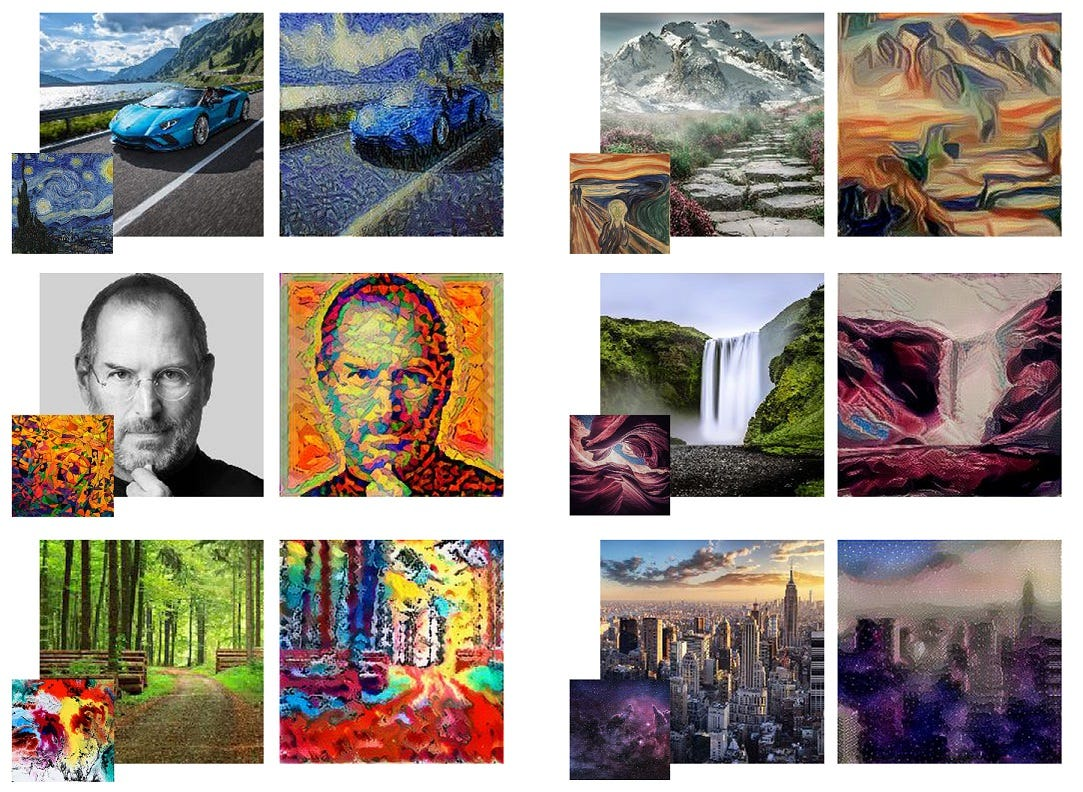This tutorial will be covering the following parts in the coming sections of the tutorial.

• Why neural style transfer and the high level architecture
• Defining inputs, outputs, losses and the optimiser for the neural style transfer network
• Defining an input pipeline to feed data to the network
• Training the network and saving the results
• Conclusion

The other articles of this series can be found below.

A B C D* E F G H I J K L* M N O P Q R S T U V W X Y Z

* denotes articles behind the Medium paywall

Aim of this article

The aim of this article is to provide a principled guide, rather than a dry run down of the algorithm, or stifling the reader with long stripes of boring code. Particularly by the end of this article, I would like the readers to grok the concepts behind NST and know why certain things are the way they are (e.g. the loss function). As an added benefit, the readers can go through the end-to-end code and see things in action.

Code

Note that I will be sharing only the most important code snippets in the article. You can get the full code as a Jupyter notebook here. The algorithm is implemented with TensorFlow.

Why NST?

Deep neural networks have already surpassed human level performance in tasks such as object recognition and detection. However, deep networks were lagging far behind in tasks like generating artistic artefacts having high perceptual quality until recent times. Creating better quality art using machine learning techniques is imperative for reaching human-like capabilities, as well as opens up a new spectrum of possibilities. And with the advancement of computer hardware as well as the proliferation of deep learning, deep learning is right now being used to create art. For example, an AI generated art won’t be sold at an auction for a whopping \$432,500.

High level architecture

As stated earlier, neural style transfer uses a pretrained convolution neural network. Then to define a loss function which blends two images seamlessly to create visually appealing art, NST defines the following inputs:

• A content image (c) — the image we want to transfer a style to
• A style image (s) — the image we want to transfer the style from
• An input (generated) image (g) — the image that contains the final result (the only trainable variable)

The architecture of the model as well as how the loss is computed is shown below. You do not need to develop a profound understanding of what is going on in the image below, as you will be seeing each component in detail in the next several sections to come. The idea is to give a high level understanding of the workflow taking place during style transfer.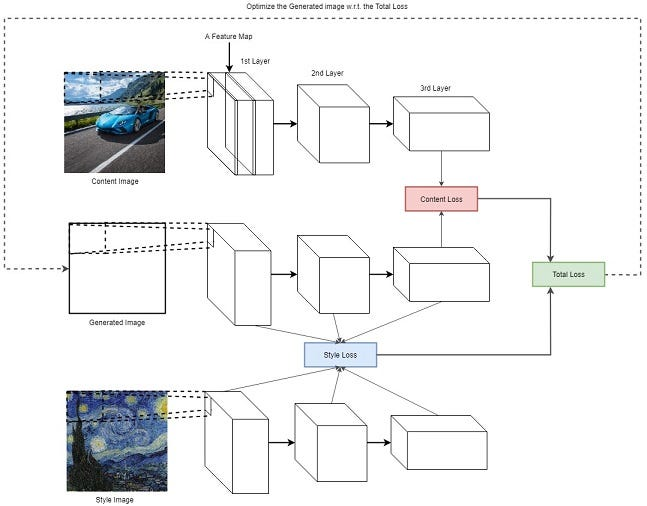High level architecture of NST model

You will be borrowing the VGG-16 weights from this webpage. You will need to download the vgg16_weights.npz file and place that in a folder called `vgg` in your project home directory (sorry I should have automated this, but I was lazy). You will only be needing the convolution and the pooling layers. Specifically, you will be loading the first `7` convolutional layers to be used as the NST network. You can do this using the `load_weights(...)` function given in the notebook.

Note: You are welcome to try more layers. But beware of the memory limitations of your CPU and GPU.

Defining functions to build the style transfer network

Here you define several functions that will help you later to fully define the computational graph of the CNN given an input.

Creating TensorFlow variables

Here you load the loaded numpy arrays into TensorFlow variables. We will be creating following variables:

• content image (`tf.placeholder`)
• style image (`tf.placeholder`)
• generated image (`tf.Variable` and `trainable=True`)
• pretrained weights and biases (`tf.Variable` and `trainable=False`)

Make sure you leave the generated image trainable while keeping pretrained weights and biases frozen. Below we show two functions to define inputs and neural network weights.

Computing the VGG net output

Here you are computing the VGG net output by means of convolution and pooling operations. Note that you are replacing the `tf.nn.max_pool` with the `tf.nn.avg_pool` operation, as `tf.nn.avg_pool` gives better visually pleasing results during style transfer . Feel free to experiment with `tf.nn.max_pool` by changing the operation in the function below.

Loss functions

In this section we define two loss functions; the content loss function and the style loss function. The content loss function ensures that the activations of the higher layers are similar between the content image and the generated image. The style loss function makes sure that the correlation of activations in all the layers are similar between the style image and the generated image. We will be discussing the details below.

Content cost function

The content cost function is making sure that the content present in the content image is captured in the generated image. It has been found that CNNs capture information about content in the higher levels, where the lower levels are more focused on individual pixel values . Therefore we use the top-most CNN layer to define the content loss function.

Let A^l_{ij}(I) be the activation of the l th layer, i th feature map and j th position obtained using the image I. Then the content loss is defined as,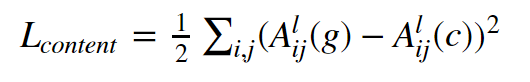The content loss

Essentially L_{content} captures the root mean squared error between the activations produced by the generated image and the content image. But why does minimising the difference between the activations of higher layers ensure the content of the content image is preserved?

Intuition behind content loss

If you visualise what is learnt by a neural network, there’s evidence that suggests that different feature maps in higher layers are activated in the presence of different objects. So if two images to have the same content, they should have similar activations in the higher layers.

We can define the content cost as follows.

Style loss function

Defining the style loss function requires more work. To extract the style information from the VGG network, we use all the layers of the CNN. Furthermore, style information is measured as the amount of correlation present between features maps in a given layer. Next, a loss is defined as the difference of correlation present between the feature maps computed by the generated image and the style image. Mathematically, the style loss is defined as,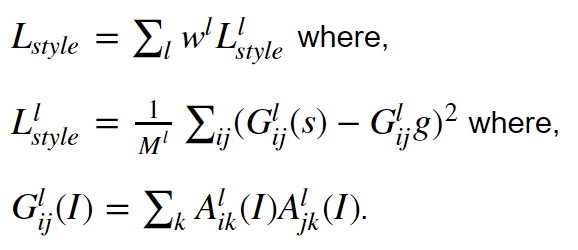w^l (chosen uniform in this tutorial) is a weight given to each layer during loss computation and M^l is an hyperparameter that depends on the size of the l th layer. If you would like to see the exact value, please refer to this paper. However in this implementation, you are not using M^l as that will be absorbed by another parameter when defining the final loss.

Intuition behind the style loss

Though the above equation system is a mouthful, the idea is relatively simple. The goal is to compute a style matrix (visualised below) for the generated image and the style image. Then the style loss is defined as the root mean square difference between the two style matrices.

Below you can see an illustration of how the style matrix is computed. The style matrix is essentially a Gram matrix, where the (i,j) th element of the style matrix is computed by computing the element wise multiplication of the i th and j th feature maps and summing across both width and height. In the figure, red cross denotes element wise multiplication and the red plus sign denotes summing across both width height of the feature maps.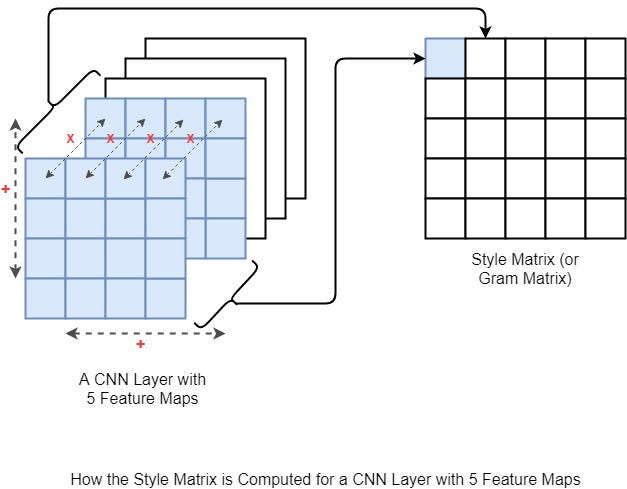You can compute the style loss as follows.

Why is it that style is captured in the Gram matrix?

It’s great that we know how to compute the style loss. But you still haven’t been shown “why the style loss is computed using the Gram matrix”. The Gram matrix essentially captures the “distribution of features” of a set of feature maps in a given layer. By trying to minimise the style loss between two images, you are essentially matching the distribution of features between the two images [3, 4].

Note: Personally, I don’t think the above question has been answered satisfactorily. For example  explains the similarities between the style loss and domain adaptation. But this relationship does not answer the above question.

So let me take a shot at explaining this a bit more intuitively. Say you have the following feature maps. For simplicity I assume only three feature maps, and two of them are completely inactive. You have one feature map set where the first feature map looks like a dog, and in the second feature map set, the first feature map looks like a dog upside down. Then if you try to manually compute content and style losses, you will get these values. This means that we haven’t lost style information between two feature map sets. However, the content is quite different.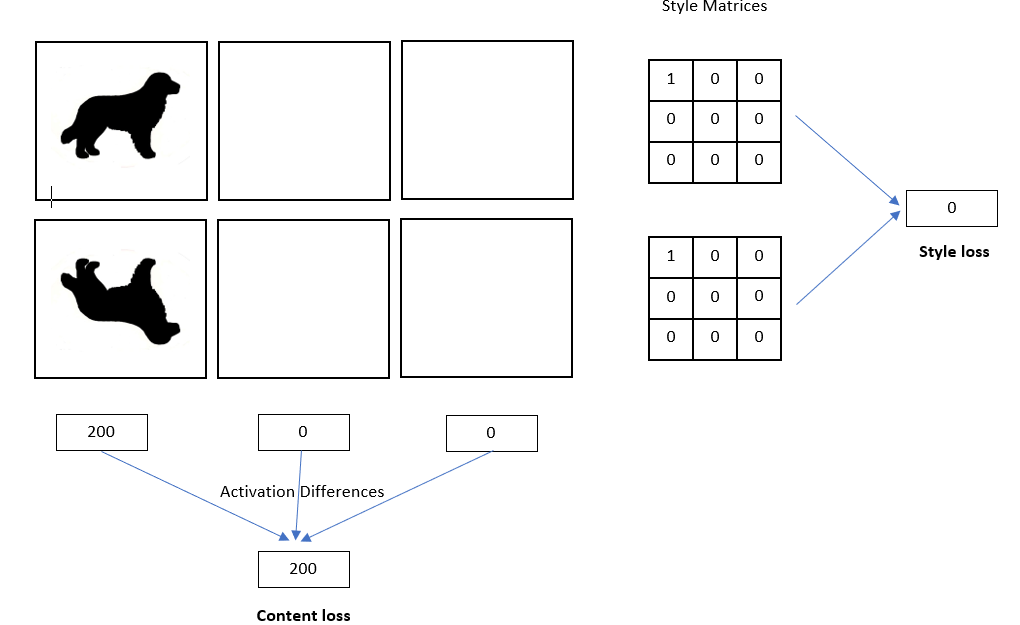Understanding style loss

Final loss

The final loss is defined as,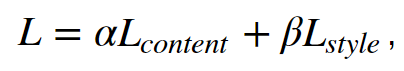where α and β are user-defined hyperparameters. Here β has absorbed the M^l normalisation factor defined earlier. By controlling α and β you can control the amount of content and style injected to the generated image. You can also see a nice visualisation of different effects of different α and β values in the paper.

Defining the optimiser

Next you use the Adam optimiser to optimise the loss of the network.

Defining the input pipeline

Here you define the full input pipeline. `tf.data` provides a very easy to use and intuitive interface to implementing input pipelines. For most of the image manipulation tasks you can use the `tf.image` API, however the ability of `tf.image` to handle dynamic sized images is very limited. For example, if you want to dynamically crop and resize images it is better to do in the form of a generator as implemented below.

You have defined two input pipelines; one for content and one for style. The content input pipeline looks for `jpg` images that start with the word `content_`, where the style pipeline looks for images starting with `style_`.

Defining the computational graph

Now you are ready to rock and roll! In this section you will be defining the full computational graph.

• Define iterators that provide inputs
• Define inputs and CNN variables
• Define the content, style and the total loss
• Define the optimisation operation

Running style transfer

Time to run the computational graph and generate some artwork. The generated artwork will be saved to `data/gen_0`, `data/gen_1`, …, `data/gen_5`, etc. folders.

When you run the above code, you should be getting some neat art saved to your disk like below.Conclusion

In this tutorial, you learnt about neural style transfer. Neural style transfer allows to blend two images (one containing content and one containing style) together to create new art. You first went through why you need neural style transfer and an overview of the architecture of the method. Then you defined the specifics of the neural style transfer network with TensorFlow. Specifically, you defined several functions to define the variables/inputs, compute the VGG output, compute the losses and perform the optimisation. You next understood the two losses that allow us to achieve what we want; the content loss and the style loss in detail, and saw how they come together to define the final loss. Finally you ran the model and saw artwork generated by the model.

Code for this tutorial is available here.